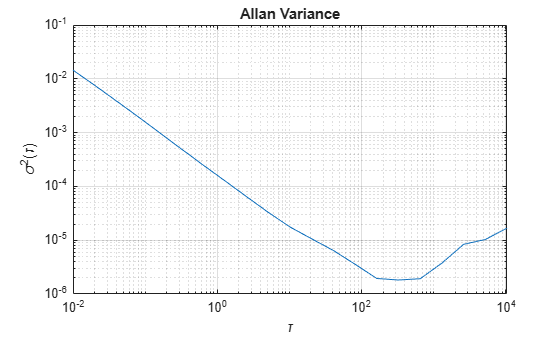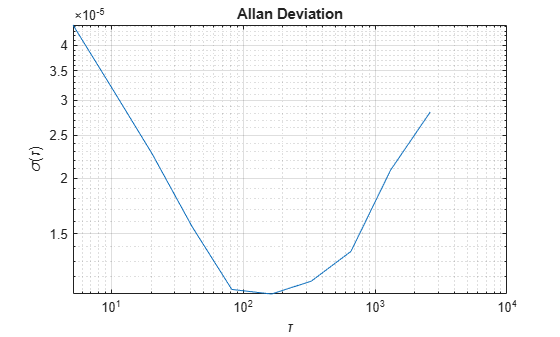allanvar

Allan variance

Description

Allan variance is used to measure the frequency stability of oscillation for a sequence of data in the time domain. It can also be used to determine the intrinsic noise in a system as a function of the averaging time. The averaging time series τ can be specified as τ = m/fs. Here fs is the sampling frequency of data, and m is a list of ascending averaging factors (such as 1, 2, 4, 8, …).

[avar,tau] = allanvar(Omega) returns the Allan variance avar as a function of averaging time tau. The default averaging time tau is an octave sequence given as (1, 2, ..., 2floor{log2[(N-1)/2]}), where N is the number of samples in Omega. If Omega is specified as a matrix, allanvar operates over the columns of omega.

[avar,tau] = allanvar(Omega,m) returns the Allan variance avar for specific values of tau defined by m. Since the default frequency fs is assumed to be 1, the output tau is exactly same with m.

[avar,tau] = allanvar(Omega,ptStr) sets averaging factor m to the specified point specification, ptStr. Since the default frequency fs is 1, the output tau is exactly equal to the specified m. ptStr can be specified as 'octave' or 'decade'.

example

[avar,tau] = allanvar(___,fs) also allows you to provide the sampling frequency fs of the input data omega in Hz. This input parameter can be used with any of the previous syntaxes.

Examples

collapse all

Load gyroscope data from a MAT file, including the sample rate of the data in Hz. Calculate the Allan variance.

[avar,tau] = allanvar(omega,'octave',Fs);

Plot the Allan variance on a loglog plot.

loglog(tau,avar)
xlabel('\tau')
ylabel('\sigma^2(\tau)')
title('Allan Variance')
grid onGenerate sample gyroscope noise, including angle random walk and rate random walk.

numSamples = 1e6;
Fs = 100;
nStd = 1e-3;
kStd = 1e-7;
nNoise = nStd.*randn(numSamples,1);
kNoise = kStd.*cumsum(randn(numSamples,1));
omega = nNoise+kNoise;

Calculate the Allan deviation at specific values of $\mathit{m}=\tau$. The Allan deviation is the square root of the Allan variance.

m = 2.^(9:18);
[avar,tau] = allanvar(omega,m,Fs);

Plot the Allan deviation on a loglog plot.

xlabel('\tau')
ylabel('\sigma(\tau)')
title('Allan Deviation')
grid onInput Arguments

collapse all

Input data specified as an N-by-1 vector or an N-by-M matrix. N is the number of samples, and M is the number of sample sets. If specified as a matrix, allanvar operates over the columns of Omega.

Data Types: single | double

Averaging factor, specified as a scalar or vector with ascending integer values less than (N-1)/2, where N is the number of samples in Omega.

Data Types: single | double

Point specification of m, specified as 'octave' or 'decade'. Based on the value of ptStr, m is specified as following:

• If ptStr is specified as 'octave', m is:

$\left[{2}^{0},{2}^{1}{...2}^{⌊{\mathrm{log}}_{2}\left(\frac{N-1}{2}\right)⌋}\right]$

• If ptStr is specified as 'decade', m is:

$\left[{10}^{0},{10}^{1}{...10}^{⌊{\mathrm{log}}_{10}\left(\frac{N-1}{2}\right)⌋}\right]$

N is the number of samples in Omega.

Basic frequency of the input data, Omega, in Hz, specified as a positive scalar.

Data Types: single | double

Output Arguments

collapse all

Allan variance of input data at tau, returned as a vector or matrix.

Averaging time of Allan variance, returned as a vector, or a matrix.